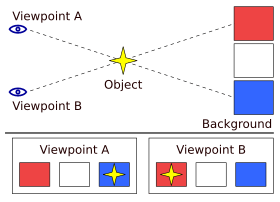# Parallax facts for kids

Kids Encyclopedia FactsAn example of the parallax of an object against a distant background due to a change in location. When viewed from "Viewpoint A", the object appears to be in front of the blue square. When the viewpoint is changed to "Viewpoint B", the object appears to have moved in front of the red square.

Parallax is the perceived change in position of an object seen from two different places.

In astronomy, annual parallax is the only direct way to measure distance to stars outside the solar system. In essence, parallax is the phenomenon which occurs when an object is viewed from different positions.

It is measured by the angle between two lines of observation. Nearby objects have a larger parallax than more distant objects when observed from identical positions, so parallax can be used to determine distances.

Astronomers use the principle of parallax to measure distances to celestial objects including to the Moon, the Sun, and to stars beyond the Solar System. Here, the term "parallax" is the angle between two sight-lines to the star.

Astronomical measurement of position are taken at different times of the year. Since the Earth's orbit is known exactly, the distance from position 1 to position 2 can be worked out. Then, the angle from the horizon to the object can be measured precisely. This gives a triangle whose baseline and angles are known accurately. From the triangle, the distance is calculated by trigonometry.

The method only fails with objects which are so distant that the long axis of the earth's orbit is too short to get a sufficient difference in the measured angle. Astronomers have worked out various ways to get over this problem, though none are so accurate as the parallax method is for relatively nearby objects. This provides a basis for the cosmic distance ladder. This is the name for the techniques astronomers use to calculate distances further than the parallax method allows.

From 1989 to 1993 the Hipparcos satellite took measurements for over 100,000 nearby stars.

Many animals, including humans, have two eyes which provide depth perception; this is called stereopsis. Because the two eyes are at different places on the head, this gives the basis for an automatic sense of distance. We perceive this as a normal 3-D scene.

## Images for kidsParallax Facts for Kids. Kiddle Encyclopedia.